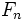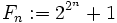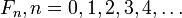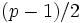Fermat number

This article describes a sequence of natural numbers. The parameter for the sequence is a positive integer (or sometimes, nonnegative integer).
View other one-parameter sequences
This article defines a property that can be evaluated for a natural number, i.e., every natural number either satisfies the property or does not satisfy the property.
View a complete list of properties of natural numbers

Definition

Letbe a nonnegative integer. TheFermat number, denoted, is defined as:.

If it is prime, it is termed a Fermat prime.

Occurrence

Initial values

The initial values ofare 3, 5, 17, 257, 65537, 4294967297 View list on OEIS

Relation with other properties

Other related properties

• Safe prime is a primesuch thatis also prime.
• Mersenne number is a number of the form, and a Mersenne prime is a Mersenne number that is also prime.